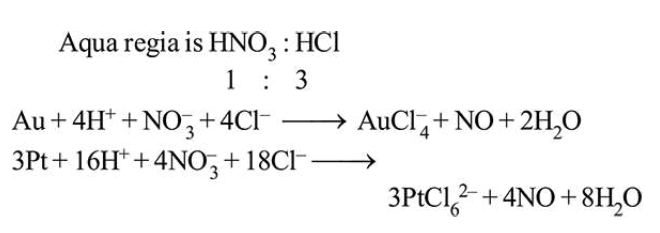# Aqua regia is used for dissolving noble metals (Au, Pt, etc.).

Question:

The gas evolved in this process is :

1. NO

2. $\mathrm{N}_{2} \mathrm{O}_{5}$

3.  $\mathrm{N}_{2}$

4. $\mathrm{N}_{2} \mathrm{O}_{3}$

Correct Option: 1

Solution: